# Math in Focus Grade 2 Chapter 11 Practice 2 Answer Key Comparing Amounts of Money

Practice the problems of Math in Focus Grade 2 Workbook Answer Key Chapter 11 Practice 2 Comparing Amounts of Money to score better marks in the exam.

## Math in Focus Grade 2 Chapter 11 Practice 2 Answer Key Comparing Amounts of Money

Compare the amounts.

Complete the tables and fill in the blanks.

Example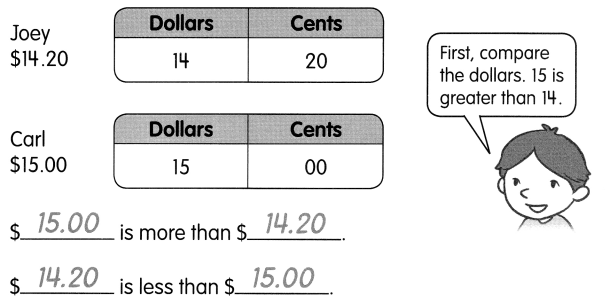Question 1.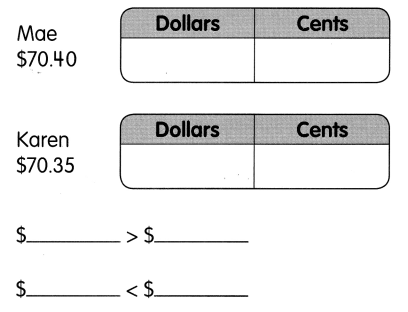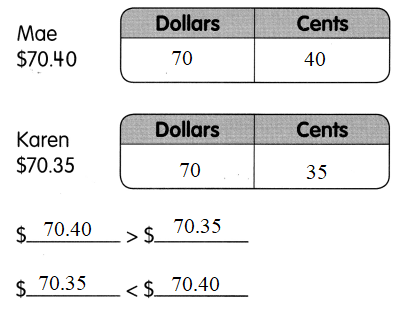$70.40 = 70 dollars and 40 cents$70.35 = 70 dollars and 35 cents
$70.40 >$70.35
$70.35 <$70.40

Question 2.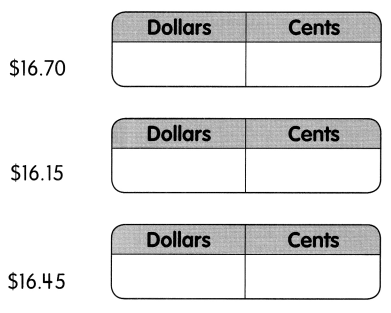Are all of these amounts the same? _______
$_________ is the greatest amount.$_________ is the least amount.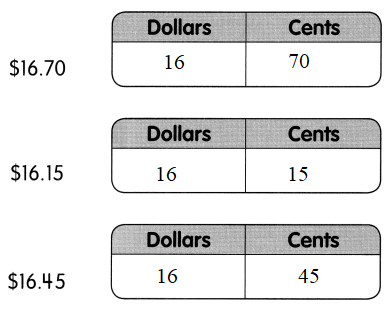$16.70 = 16 dollars and 70 cents.$16.15 = 16 dollars and 15 cents.
$16.45 = 16 dollars and 45 cents. All the amounts are not same$16.70 is the greatest amount.
$16.15 is the least amount. Question 3.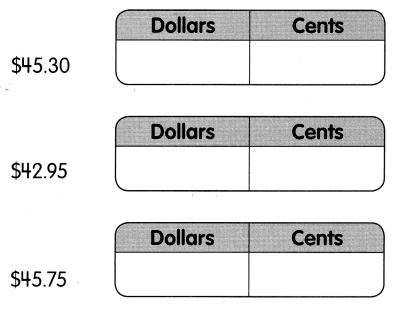Which is the greatest amount? _________ Which is the least amount? _________ Answer: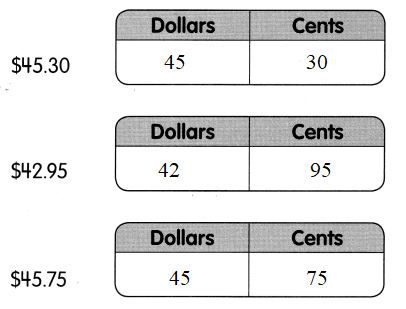$45.30 = 45 dollars and 30 cents.
$42.95 = 42 dollars and 95 cents.$45.75 = 45 dollars and 75 cents.
$45.75 is the greatest amount.$42.95 is the smallest amount.

Write the amount in each set.
Then check (✓) the set that has the greater value.

Example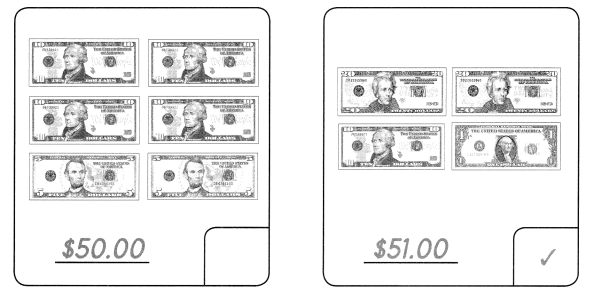Question 4.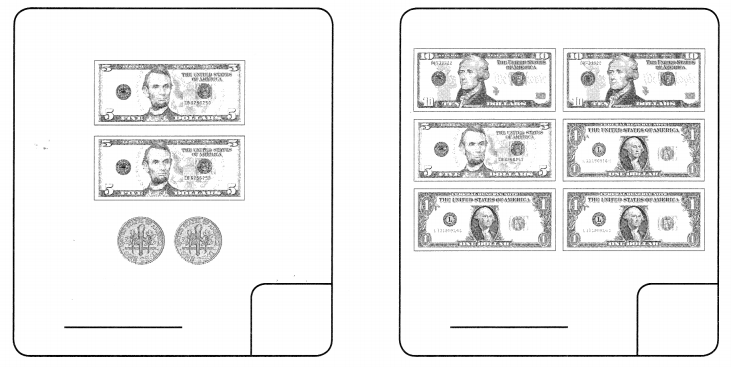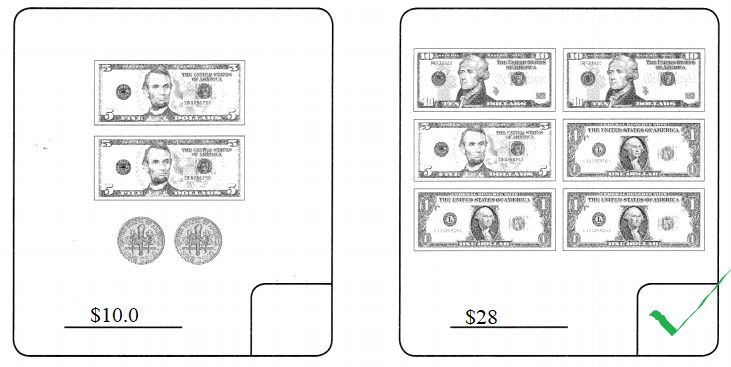5 dollars + 5 dollars + 1 dime + 1 dime = 10 dollars + 2 dime
1 dime = 0.1dollar
so 10.1 dollar
10 dollars + 10 dollars + 5 dollars + 1 dollar + 1 dollar + 1 dollar = 28 dollars

Question 5.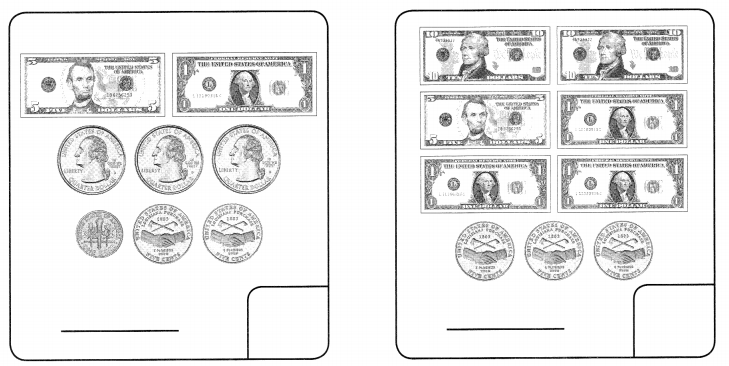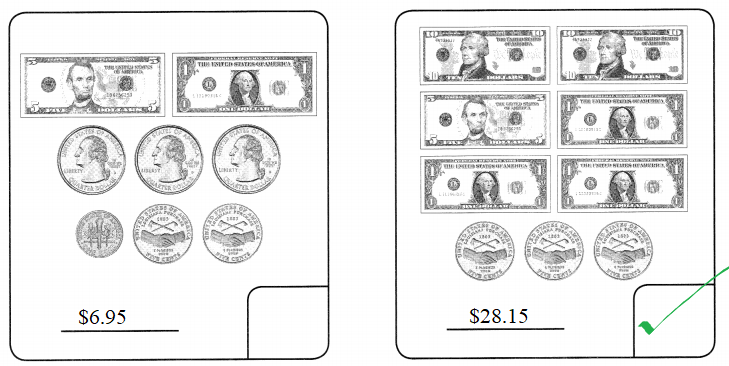5 dollars + 1 dollar + quarter dollar + quarter doller + quarter dollar + 1 dime + 5 cents + 5 cents
Quarter dollar = 25 cents.
6 dollars 85 cents 1 dime.
1 dime = 10cents
85 cents 1 dime = 95 cents
so
$6.95 10 dollars + 10 dollars + 5 dollars + 1 dollar + 1 dollar + 1 doller + 5 cents + 5 cents + 5 cents = 28 dollars 15 cents$28.15

Circle the amount that is less.

Question 6.
$3.85$4.10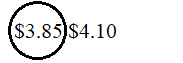$3.85 amount is less. Question 7.$62.40 $62.25 Answer: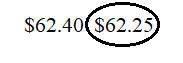$62.25 amount is less.

Circle the amount that is more.

Question 8.
$28.90$27.95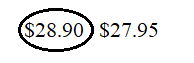$28.90 amount is more. Question 9.$71.09 $790 Answer: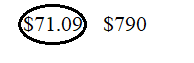$71.09 amount is more.

Compare the amounts.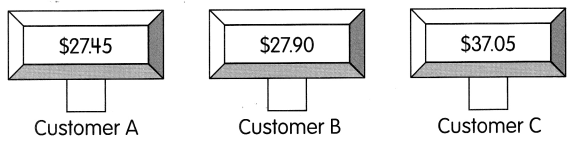$37.05 greater than$27.90 greater than \$27.45.